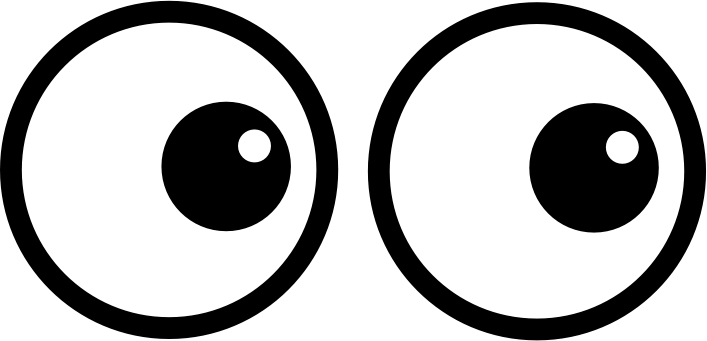# GEOM 2 | Lesson 4 | Watch (Centers of a Triangle)# Centers of a Triangle

Watch the following video that discusses properties of triangles.  Return to this page by using the back arrow.

Centers of a Triangle by Shmoop

Summarize the three centers of triangles, how they are found, and what the mathematical relationships are for each.

How do you think these centers would be related in a equilateral triangle and why?